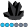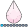## loop through rows/observations

Hello,

I need the same functionality as cursors on SQL servers. Is it possible in SAS?
Basically I want iterate through dataset and in each iteration I want to access the variables on particular row.
Can someone post here some simple solution?

1 ACCEPTED SOLUTION

Accepted Solutions

## Re: loop through rows/observations

If I'm understanding what you want: sum some value across all rows in you table.

then I think this will work.

``````data example;
set example;
if _n_ = 1 then sum=0; *I do not think you need this line but I put in for example;

sum + height; increments sum by value of height;

run;``````

I'm not sure of what you are thinking about when you say use sum function over height column. The sum function works on columns in a single row ie
something like this.... sum = sum(ht1, ht2, ht3).

This syntax sums across rows. So when i = linescount, Height will be the sum of height over all lines.

You certainly can get more creative with what you increment - as you say some more complex calculation

for example : sum + (a + b*(c^2)*d/e); where a-e are all columns.

Hope this helps

17 REPLIES 17

## Re: loop through rows/observations

Hi:
I'm not exactly sure what 'cursors' does in SQL or what you are trying to achieve with cursors. Can you post some code that uses cursors, or better, describe your data and what it is you need to do?

I do note that in the documentation:
http://support.sas.com/documentation/cdl/en/acreldb/63647/HTML/default/viewer.htm#a001371529.htm

There is a statement about the CURSOR_TYPE= dataset option that
"Not all drivers support all cursor types. An error is returned if the specified cursor type is not supported." So, if you are using SAS/Access for a relational database, you might find the CURSOR_TYPE dataset option useful to investigate.

cynthia

## Re: loop through rows/observations

Dear Cynthia,

Basically using cursors you can iterate observations one by one and access each object separately.
For example you have the table with columns (Name, Heigth, Weight) and some sample data
Joe 175 70
Linda 162 55
Lucy 172 66

and i need to access each line such as below:
FOR I = 0 to DATASET.LINESCOUNT
DATASET(I).Height ...etc
END FOR
So in each iteration i can access one line. The advantage is, that you can do any complicated calculations which cannot be done by sas functions. Disadvantage is poor performance.

When you look at this example http://www.sqlteam.com/article/cursors-an-overview
Here is the usage of cursor on microsoft sql server. In each iteration (WHILE loop), the value in row is stored to variable @AuthorID. Of course there can be more then one value. And this is what I need.

Thanks for any ideas

## Re: loop through rows/observations

Out of curiousity what type of process would a SQL cursor implemented in SAS be more efficient than a SAS datastep?

## Re: loop through rows/observations

In oracle, I can say:

for h in (select id from tbl where ....) loop
...
any processing needed for h.id, updating, deleting from other tables, sending notifications, aggregate data from other tables, etc. It essentially lets me do multiple and/or conditional data steps/sql blocks from within a data step.
...
end loop

In SAS, you would have to (or at least the best way I've figured out how to):
1. create a dataset tbl with a monotonic column (say rownum) using proc sql with monotonic() or a data step with the _N_ auto variable
2. count how many rows where in the table and store it in a macro variable (say %totrows)
3. build a macro to run a loop and use proc sql to get variables;
...
%do i = 1 to %totrows;
proc sql;
select id into :id from tbl where .... and rownum = &i;
---
any processing needed for that id
...
'%end;chang_y_chung_hotmail_com
Obsidian | Level 7

## Re: loop through rows/observations

...

> In SAS, you would have to (or at least the best way

> I've figured out how to):

> 1. create a dataset tbl with a monotonic column (say

> rownum) using proc sql with monotonic() or a data

> step with the _N_ auto variable

> 2. count how many rows where in the table and store

> it in a macro variable (say %totrows)

> 3. build a macro to run a loop and use proc sql to

> get variables;

> ...

> %do i = 1 to %totrows;

> proc sql;

> select id into :id from tbl where .... and rownum =

> &i;

> ---

> any processing needed for that id

> ...

> '%end;

:-)

## Re: loop through rows/observations

Can you please advise how one can output the contents of the PDV for each iteration as one steps through a SAS Program in order to validate the logic on a test case?chang_y_chung_hotmail_com
Obsidian | Level 7

## Re: loop through rows/observations

...

> I need the same functionality as cursors on SQL

> servers. Is it possible in SAS?

> Basically I want iterate through dataset and in each

> iteration I want to access the variables on

> particular row.

...

Is it possible in SAS?! You are kidding, right? 🙂

Data step is for iterating over observations. A simple data step with a set statement is rather exactly like using a forward (only) cursor without much fuss (iteration is built in):

data _null_;

set sashelp.class;

where sex = "M";

put name= age= sex=;

run;

/* on log

Name=Alfred Age=14 Sex=M

Name=Henry Age=14 Sex=M

Name=James Age=12 Sex=M

Name=Jeffrey Age=13 Sex=M

Name=John Age=12 Sex=M

Name=Philip Age=16 Sex=M

Name=Robert Age=12 Sex=M

Name=Ronald Age=15 Sex=M

Name=Thomas Age=11 Sex=M

Name=William Age=15 Sex=M

*/

## Re: loop through rows/observations

Hi,

Thank you for reply, I am not kidding. I am sql/.net developer forced to work with sas and a lot of routine operations which I was used to work with, doesn't work in sas.

It is nice, that iteration is built in, but i need to access each variable in row/obseravation separately and make computation over it. Please see the post I have replied to Cynthia. There is exactly what I would like to do.

ThanksKsharp
Super User

## Re: loop through rows/observations

It looks like you need SAS " array{*} " statement.Tim_SAS
Barite | Level 11

## Re: loop through rows/observations

It sounds like you're looking for the POINT= option on the SET statement.art297
Opal | Level 21

## Re: loop through rows/observations

Julo,

I think that Cynthia put you in the right direction already. I'm sure that you'll have to do a number of Google searches to find all of the info you want, but another place to start might be:
http://support.sas.com/documentation/tools/oledb/app_cursorlocktypes.htm

HTH,
Art

## Re: loop through rows/observations

I'm not sure if I'm missing something here on what you want to do but we use cursors in oracle/sql and I have not seen anything so complicated that a SAS data step cannot accomplish the same thing.

The data step inputs a single record, acts upon the objects (vars) of that record and then outpts that record. It then retrieves the next record, (if there is one) and repeats the process.

FOR I = 0 to DATASET.LINESCOUNT
DATASET(I).Height ...etc
END FOR

The data step equivelent is
data example;
set example;
height = .......;
run;

The for/end for is implied in the data step. The specific line can be reference by automatic variable (linescount= _n_;

This will iterate through each row of your example table and perform whatever operation you want on height. This is very simplified but you could get pretty complex in what you do with that observation.

Of course it would have been helpful if an example of what exactly you do with sql/cursor was provided. What specific operations that you "used to work with, doesn't work in sas".

## Re: loop through rows/observations

Hi LAP,

Thanks - i had no idea about referencing specific line. I have solved my problem using proc iml, but better (or more clear) solution is to use data step.

For example - if i want to make a sum of height variable from my previous example.
INT SUM = 0;.
FOR I = 0 to DATASET.LINESCOUNT
SUM = SUM + DATASET(I).Height.
END FOR

How can I do this in data step? Declare variable (not column in table) out of the data step.

sum = 0;
data example;
set example;
sum = sum + height
run;

Please do not answer something like : use sum function over height column 🙂
This is only an example, the calculation will be more complex.

## Re: loop through rows/observations

when IML can solve your problem, it will probably be more effective than a data step when you want differing calculations down different subsets and different columns, because iml allows you to load the whole table into an array (matrix). Syntax and processing refer only to the copy in memory. So that is also the limitation of the IML approach - Some data sets will be too big for memory (unless you are very very well resourced - not many of us have memory for a 100gb matrix) .

peterC
Discussion stats
• 17 replies
• 80070 views
• 1 like
• 11 in conversation